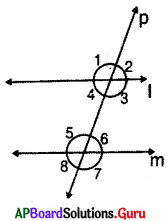Practice the AP 7th Class Maths Bits with Answers 4th Lesson Lines and Angles on a regular basis so that you can attempt exams with utmost confidence.

## AP Board 7th Class Maths Bits 4th Lesson Lines and Angles

Multiple Choice Questions :

Question 1.
Union of two rays which has one com¬mon end point is called ________
(A) Line
(B) Ray
(C) Angle
(D) Triangle
(C) Angle

Question 2.
If the sum of two angles is 180°, then the angles are called ________
(A) Supplementary
(B) Complementary
(C) Conjugate
(A) Supplementary

Question 3.
If the sum of two angles is 90°, then the angles are called ________
(A) Supplementary
(B) Complementary
(C) Conjugate
(B) Complementary

Question 4.
If the sum of ∠P and ∠Q is 360°, then they are called ________
(A) Supplementary
(B) Complementary
(C) Conjugate
(C) ConjugateQuestion 5.
Supplementary angle of 30° is ________
(A) 180°
(B) 150°
(C) 60°
(D) 90°
(B) 150°

Question 6.
If the ratio of supplementary angles is 2 : 3, then the angles are ________
(A) 72°, 108°
(B) 60°, 120°
(C) 50°, 130°
(D) 40°, 140°
(A) 72°, 108°

Question 7.
Sum of pair of adjacent angles is 180°, then the angles are called ________
(A) Supplementary
(B) Complementary
(C) Linear pair
(C) Linear pair

Question 8.
A linear pair of angles must be ________ angles.
(A) Supplementary
(B) Complementary
(D) Linear pair

Question 9.
The sum of the angles at a point on the same side of the line is ________
(A) 90°
(B) 360°
(C) 270°
(D) 180°
(D) 180°

Question 10.
Linear pair among the following :
(A) 98°, 102°
(B) 120°, 60°
(C) 180°, 90°
(D) 120°, 150°
(B) 120°, 60°

Question 11.
Vertically opposite angles are
(A) equal
(C) linear pair
(D) not equal
(A) equal

Question 12.
A line which intersects the two or more straight lines at distinct points is called a / an ________
(A) Angle
(B) Parallel line
(C) Transversal line
(D) Intersecting line
(C) Transversal line

Question 13.
Angles in between two parallel lines are called ________
(A) corresponding angles
(B) alternate interior angles
(C) exterior angles
(D) interior angles
(D) interior anglesQuestion 14.
Two angles which are on the same side of transversal, one interior and other exterior but not. adjacent are called ________
(A) corresponding angles
(B) alternate interior angles
(C) alternate exterior angles
(D) interior angles
(A) corresponding angles

Question 15.
Exterior angles oil either side of the transversal but not adjacent angles are called ________
(A) corresponding angles
(B) alternate interior angles
(C) alternate exterior angles
(D) interior angles
(C) alternate exterior angles

Question 16.
Interior angles on same side of a transversal are called ________
(A) corresponding angles
(B) interior angles
(C) co-exterior angles
(D) co-interior angles
(D) co-interior angles

Question 17.
If a transversal intersects two lines in such way that the pair of correspond¬ing angles are equal, then the two lines are ________
(A) intersecting lines
(B) parallel lines
(C) transversal lines
(D) none
(B) parallel lines

Question 18.
When the co-interior angles are supplementary, then the lines are ________
(A) intersecting lines
(B) parallel lines
(C) transversal lines
(D) none
(B) parallel lines

Question 19.
Examples for linear pair of angles in our surroundings ________
(A) pen stand
(B) electrical pole
(C) tree
(D) all of the above
(D) all of the above

Question 20.
Number of transversal lines can be drawn two distinct lines are ________
(A) 1
(B) 2
(C) infinite
(D) none
(C) infinite

Question 21.
From the adjacent figure ∠p = ________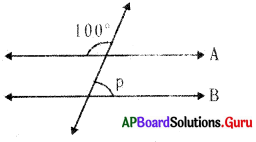(A) 100°
(B)70°
(C) 80°
(D) 60
(C) 80°

Question 22.
From the given figure ∠x =________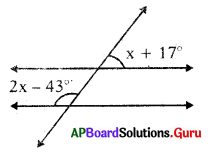(A) 60°
(B) 50°
(C) 70°
(D) 90°
(A) 60°

Question 23.
If two lines intersect each other, then the number of common points they have ________
(A) 1
(B) 2
(C) 4
(D) infinity

Question 24.
Which of the following angle can’t be formed by a normal scissors?
(A) obtuse angle
(B) acute angle
(C) right angle
(D) straight angle
(A) 1Question 25.
In the following figure,
∠x + ∠z = ________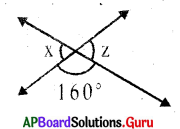(A) 20°
(B) 40°
(C) 160°
(D) 80°
(B) 40°

Fill in the blanks :

Question 1.
If the sum of two angles is 360°, then the angles are called ________
Conjugate angles

Question 2.
If the sum of ∠A and ∠B is 180°, then they are called ________
Supplementary angles

Question 3.
If the sum of ∠X and ∠Y is 90°, then they are called ________
Complementary angles

Question 4.
Conjugate angle of 120° is ________
240°

Question 5.
Complementary angle of 40° is ________
50°

Question 6.
In the ratio of complementary angles is 4 : 5, then the angles are ________
40°, 50°

Question 7.
If ∠X and ∠Y are supplementary then ________
90°

Question 8.
If the two angles have a common vertex, common arm and lie on either side of the common arm they are called ________

Question 9.
Adjacent angles need not be ________
complementary or supplementaryQuestion 10.
Linear pair of angles are ________
supplementary

Question 11.
Adjacent angles need, not be ________
linear pair

Question 12.
The sum of all the angles at a point is ________
360°

Question 13.
When two straight lines intersect each other, the pair of opposite angles formed at the point of intersection are called ________
vertically opposite angles

Question 14.
Angles that are not in between two parallel lines are called ________
exterior angles

Question 15.
Interior angles on either side of the transversal but not adjacent angles are called ________
alternate interior angles

Question 16.
Exterior angles on same side of transversal are called ________
Co-exterior angles

Question 17.
Two coplanar lines which do not intersect at any point are called as ________
parallel lines

Question 18.
When a transversal intersect a pair of parallel lines, then the alternate interior angles are ________
equal

Question 19.
If the alternate interior angles are equal, then the lines are ________
parallel

Question 20.
In the figure the value of x is ________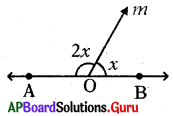60°

Question 21.
In the figure ∠2 = ∠5, then the lines are ________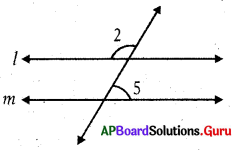intersecting linesQuestion 22.
Supplementary of an angle is three times the angle, then the angle is
60°

Question 23.
From the given figure ∠a: ∠b = 2: 1, then ∠c – ∠d = ________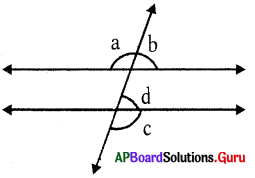60°

Question 24.
A transversal line intersects two distinct lines in ________ distinct points.
two

Question 25.
An angle is double of its supplement, then the measure of the angle is ________
60°

Match the following :

Question 1.

 Group A Group B 1. Sum of Complementary angles (A) 45° 2. Sum of Conjugate angles (B) 90° 3. Sum of Supplementary angles (C) 150° 4. Acute angle (D) 180° 5. Obtuse angle (E) 360°

 Group A Group B 1. Sum of Complementary angles (B) 90° 2. Sum of Conjugate angles (E) 360° 3. Sum of Supplementary angles (D) 180° 4. Acute angle (A) 45° 5. Obtuse angle (C) 150°

Question 2.

 Group A Group B 1. Supplementary angle of 30° is (A) 240° 2. Complementary angle of 45° is (B) 130° 3. Conjugate of 120° is (C) 70° 4. Linear pair of 50° is (D) 150° 5. the Corresponding angle of 70° is (E) 45°

 Group A Group B 1. Supplementary angle of 30° is (D) 150° 2. Complementary angle of 45° is (E) 45° 3. Conjugate of 120° is (A) 240° 4. Linear pair of 50° is (B) 130° 5. the Corresponding angle of 70° is (C) 70°Question 3.